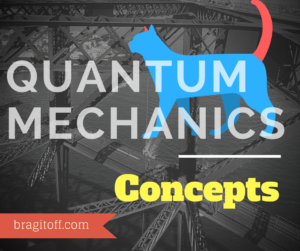# Hellman-Feynman Theorem and its Applications – Quantum MechanicsHellman-Feynman Theorem investigates how the energy of a system varies as the Hamiltonian varies.
If a system is characterised by a Hamiltonian$H$ that depends on a parameter$\lambda$, then the Hellmann-Feynman theorem states that,$\left<\frac{\partial H}{\partial \lambda}\right>=\frac{d E_n}{d \lambda}$

where,$E_n$ is the energy of the system.

### Proof:$H\left|\psi_n\right>=E_n\left|\psi_n\right>$$\implies \left<\psi_n\right|H\left|\psi_n\right> = \left<\psi_n\right|E_n\left|\psi_n\right>$$\implies \left<\psi_n\right|H\left|\psi_n\right> = E_n\left<\psi_n|\psi_n\right>$

Since the wavefunctions must be normalised,$\implies \left<\psi_n\right|H\left|\psi_n\right> = E_n$

Differentiating both sides w.r.t some parameter$\lambda$$\implies \frac{d }{d \lambda}\left<\psi_n\right|H\left|\psi_n\right> = \frac{d E_n}{d \lambda}$$\implies \left<\frac{\partial \psi_n}{\partial \lambda}|H|\psi_n\right>+\left<\psi_n|\frac{\partial H}{\partial \lambda}|\psi_n\right>+ \left<\psi_n|H|\frac{\partial \psi_n}{\partial \lambda}\right> = \frac{d E_n}{d \lambda}$

Since,$H\left|\psi_n\right>=E_n\left|\psi\right>$,$\implies E_n\left<\frac{\partial \psi_n}{\partial \lambda}|\psi_n\right>+\left<\psi_n|\frac{\partial H}{\partial \lambda}|\psi_n\right>+ E_n\left<\psi_n|\frac{\partial \psi_n}{\partial \lambda}\right> = \frac{d E_n}{d\lambda}$$\implies \left<\psi_n|\frac{\partial H}{\partial \lambda}|\psi_n\right>+E_n\left<\frac{\partial \psi_n}{\partial \lambda}|\psi_n\right>+E_n\left<\psi_n|\frac{\partial \psi_n}{\partial \lambda}\right> = \frac{d E_n}{d \lambda}$

The 2nd and 3rd terms on the L.H.S. can be replaced by$E_n\frac{d}{d \lambda}\left<\psi_n|\psi_n\right>$$\implies \left<\psi_n|\frac{\partial H}{\partial \lambda}|\psi_n\right> + E_n\frac{d}{d \lambda}\left<\psi_n|\psi_n\right> = \frac{d E_n}{d \lambda}$

Since$\left<\psi_n|\psi_n\right>=1$(Normalisation condition)$\implies \left<\psi_n|\frac{\partial H}{\partial \lambda}|\psi_n\right>= \frac{ d E_n}{d \lambda}$$\implies \left<\frac{\partial H}{\partial \lambda}\right>=\frac{d E_n}{d \lambda}$

### Applications:

• Expectation value of 1/r :$\left<\frac{1}{r}\right>$ for Hydrogen, using Hellmann-Feynam Theorem:

Hamiltonian:$H=\frac{p^2}{2m} - \frac{e^2}{4\pi\epsilon_0 r} + \frac{\hbar^2}{2m}\frac{l(l+1)}{r^2}$

and  Energy:$E_n=-\frac{m}{2\hbar^2}\left(\frac{e^2}{4\pi\epsilon_0}\right)^2\frac{1}{n^2}$

Taking,$\lambda=e$, in the Hellmann-Feynman theorem,$\left<\frac{\partial H}{\partial e}\right>=\frac{d E_n}{d e}$$\implies -\left<\frac{2e}{4\pi\epsilon_0 r}\right> = -\frac{m}{2\hbar^2}\frac{4e^3}{(4\pi\epsilon_0)^2}\frac{1}{n^2}$$\implies \frac{2e}{4\pi\epsilon_0}\left<\frac{1}{r}\right> = \frac{m}{2\hbar^2}\frac{4e^3}{(4\pi\epsilon_0)^2}\frac{1}{n^2}$$\implies \left<\frac{1}{r}\right> = \frac{m}{\hbar^2}\frac{e^2}{4\pi\epsilon_0}\frac{1}{n^2}$

Since, Bohr radius,$a_0= \frac{4\pi\epsilon_0\hbar^2}{m e^2}$

Therefore,$\implies \left<\frac{1}{r}\right> = \frac{1}{a_0 n^2}$

• Expectation value of 1/r^2 :$\left<\frac{1}{r^2}\right>$ for Hydrogen, using Hellmann-Feynam Theorem:

Hamiltonian:$H=\frac{p^2}{2m} - \frac{e^2}{4\pi\epsilon_0 r} + \frac{\hbar^2}{2m}\frac{l(l+1)}{r^2}$

and  Energy:$E_n=-\frac{m}{2\hbar^2}\left(\frac{e^2}{4\pi\epsilon_0}\right)^2\frac{1}{(j_{max}+l+1)^2}$

Taking,$\lambda=l$, in the Hellmann-Feynman theorem,$\left<\frac{\partial H}{\partial l}\right>=\frac{d E_n}{d l}$

• Harmonic Oscillator:

Relation between$\left$ and$E_n$:
Take$\lambda=\omega$

Relation between$\left$ and$E_n$:
Take$\lambda=\hbar$

Relation between$\left$ and$\left$:
Take$\lambda=m$[wpedon id="7041" align="center"]